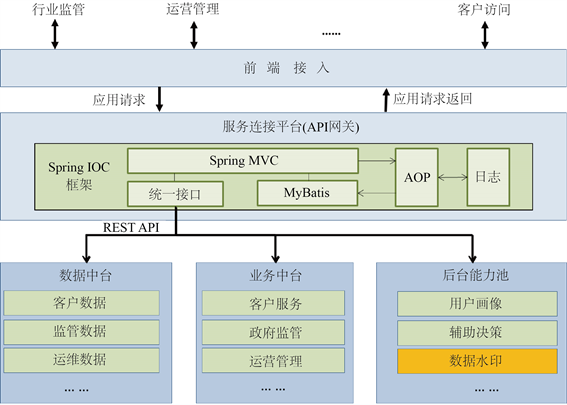﻿ 基于自适应嵌入的高抗毁数据水印技术研究

# 基于自适应嵌入的高抗毁数据水印技术研究Research on High Invulnerability Data Watermarking Technology Based on Adaptive Embedding

Abstract: Under the National big data strategy, data needs to be transferred, reorganized and used among every chain. At the same time, the management control of data is also transferred. Once the data is leaked, the data provider hopes to be able to accurately trace whether it is the responsibility of the data use link. Data watermarking technology can effectively meet the needs of data security circulation by highly imitating the real data content and adding identification information, which is not easy to be detected by data users, and so data watermarking technology has strong concealment and invulnerability. This paper proposes a high invulnerability data watermarking method based on adaptive embedding, which can flexibly face to the characteristics of the target data, and then ensure the embedding rate of the data watermark as much as possible and the availability of the target data, and also improve the invulnerability of the data watermark.

1. 引言

2. 相关知识

2.1. 概述

2.2. 技术现状

2002年Agrawal和Kiernan首次提出数据库水印的概念，以此来标记哪些是数值型的数据 。随着数据水印技术的发展，总的来说，大致可分为失真和无失真的两类水印 。数据水印的嵌入首先应该根据不同业务的差异化需求，尽量保证数据的可用性；其次数据水印应该具有完整唯一标识，保证数据泄露时溯源的准确性；最后数据水印应该具有很强的隐蔽性和抗毁性，保证数据在流转过程中，水印不会被攻击破坏。为了同时实现上述三个目标，国内外学者提出多种数据水印方法。一种思路是基于差异扩展的数据水印，同时考虑失真约束，控制数据水印的嵌入率，例如Bhattacharya和Cortesi提出的将元组划分置换后嵌入水印方法 。为了优化嵌入效果，有学者提出了遗传算法和直方图移位算法 ，但是前期计算量非常大。

3. 算法设计

3.1. 自适应分组

${A}_{g}=\left\{{x}_{i,j}|\text{if}\text{\hspace{0.17em}}hash\left({k}_{i}\right)\mathrm{mod}\left(\tau \cdot {2}^{m}\right)=g\right\}$ (3.1)

$\left\{{x}_{1,j},{x}_{2,j},\cdots ,{x}_{n,j}\right\}\to \left\{{x}_{p\left(1\right),j},{x}_{p\left(2\right),j},\cdots ,{x}_{p\left(n\right),j}\right\}$ (3.2)

${C}_{m}=\underset{t=1}{\overset{\tau }{\sum }}\frac{\frac{\underset{{x}_{i,j}\in A{A}_{t}}{\sum }{\left(p\left(i\right)-\stackrel{¯}{P}\right)}^{2}}{|A{A}_{t}|}}{t},\text{}其中A{A}_{t}=\left\{{A}_{g}|⌊\frac{g}{{2}^{m}}⌋=t\right\}$ (3.3)

${S}_{w}=数据水印位数×{2}^{m},\text{\hspace{0.17em}}\text{if}\text{\hspace{0.17em}}\mathrm{max}\left\{{C}_{m},\text{\hspace{0.17em}}m=0,1,2,\cdots \right\}$ (3.4)

${S}_{w}$ 即为自适应分组算法的分组结果，该分组方式能够保证水印在数据库中的分布更加均匀和离散，能够有效抵抗删除、更新等攻击。

3.2. 自适应嵌入强度

${E}_{j}=\frac{\underset{i=1}{\overset{n}{\sum }}{x}_{ij}}{n}$ (3.5)

${E}_{j}$ 表示第j列属性值的均值，n表示第j列属性列的属性个数。显然，均值越大，我们的修改量也可以更大，且不引起明显失真。

${D}_{j}=\frac{\underset{i=1}{\overset{n}{\sum }}{\left({x}_{ij}-{E}_{j}\right)}^{2}}{n}$ (3.6)

${D}_{j}$ 表示第j列属性值的方差，该值是用来判断该列的数值抖动情况，如果 ${D}_{j}$ 的值较小，说明该列数值变化平稳，为了避免明显失真，应使修改量较小；反之，可以嵌入较大容量的水印信息。

${e}_{j}=\left\{\begin{array}{l}1,\text{\hspace{0.17em}}\text{\hspace{0.17em}}\text{\hspace{0.17em}}\text{\hspace{0.17em}}\text{if}\text{\hspace{0.17em}}{E}_{j}\in \left[0,{g}_{1}\right)\\ 2,\text{\hspace{0.17em}}\text{\hspace{0.17em}}\text{\hspace{0.17em}}\text{if}\text{\hspace{0.17em}}{E}_{j}\in \left[{g}_{1},\infty \right)\end{array}$${d}_{j}=\left\{\begin{array}{l}1,\text{\hspace{0.17em}}\text{\hspace{0.17em}}\text{\hspace{0.17em}}\text{\hspace{0.17em}}\text{if}\text{\hspace{0.17em}}{D}_{j}\in \left[0,{g}_{2}\right)\\ 2,\text{\hspace{0.17em}}\text{\hspace{0.17em}}\text{if}\text{\hspace{0.17em}}{D}_{j}\in \left[{g}_{2},\infty \right)\end{array}$ (3.7)

${t}_{j}=\mathrm{min}\left\{{e}_{j},{d}_{j}\right\}$ (3.8)

${t}_{j}$ 为最终确定的嵌入强度等级，为保证数据失真较小，我们取均值条件下和方差条件下较低的那一个等级作为最终的强度等级。

3.3. 数据水印提取

${l}_{i,j}=\left\{\begin{array}{l}\text{error},\text{if}\text{\hspace{0.17em}}\mathrm{mod}\left({x}_{i,j},Q\right)\ne 0;\\ 0,\text{if}\text{\hspace{0.17em}}\mathrm{mod}\left({x}_{i,j},Q\right)=0\text{and}\mathrm{mod}\left(\frac{{x}_{i,j}}{Q},2\right)=0;\\ 1,\text{if}\text{\hspace{0.17em}}\mathrm{mod}\left({x}_{i,j},Q\right)=0\text{and}\mathrm{mod}\left(\frac{{x}_{i,j}}{Q},2\right)=1;\end{array}$ (3.9)

$S{T}_{\lambda }=\frac{\underset{\mathrm{mod}\left(Hash\left({k}_{i}\right),{S}_{l}\right)=\lambda }{\sum }{l}_{i,j}}{|\mathrm{mod}\left(Hash\left({k}_{i}\right),{S}_{l}\right)=\lambda |}$ (3.10)

${L}_{\lambda }=\left\{\begin{array}{l}0,\text{if}\text{\hspace{0.17em}}S{T}_{\lambda }<标识位为\text{ }\text{ }0\text{ }\text{ }的判断阈值\\ 1,\text{}\text{ }\text{ }\text{if}\text{\hspace{0.17em}}S{T}_{\lambda }>标识位为\text{ }\text{ }1\text{ }\text{ }的判断阈值\end{array}$ (3.11)

4. 软件设计及应用结果分析

4.1. 微服务能力设计Figure 1. Design of micro-services for data watermark traceability

4.2. 模拟验证

(1) 验证环境Table 1. Software and hardware verification environment

(2) 模拟样本数据Table 2. Some verification sample data

(3) 水印添加及溯源结果Table 3. Data watermark tracing results

5. 总结

 中共中央国务院. 关于构建更加完善的要素市场化配置体制机制的意见[N]. 新华社北京, 2020-4-9(电).

 张衠. 大数据安全风险与对策研究——近年来大数据安全典型事件分析[J]. 信息安全与通信保密, 2017(6): 102-107.

 张晓娟, 王文强, 唐长乐. 中美政府数据开放和个人隐私保护的政策法规研究[J]. 情报理论与实践, 2016, 39(1): 38-43.

 安小米, 穆勇, 王薇,等. 我国涉及隐私的个人信息保护与管理法律法规状况及要求分析[J]. 北京档案, 2011(5): 19-21.

 王铮, 曾萨, 安金肖, 等. 欧盟《一般数据保护条例》指导下的数据保护官制度解析与启示[J]. 图书与情报, 2018(5): 119-125.

 中华人民共和国网络安全法(2016年11月7日第十二届全国人民代表大会常务委员会第二十四次会议通过)[EB/OL]. http://www.npc.gov.cn/wxzl/gongbao/2017-02/20/content_2007531.htm, 2017-02-20.

 中华人民共和国司法部.《数据安全管理办法(征求意见稿)》[EB/OL] http://www.moj.gov.cn/news/c-ontent/2019-05/28/zlk_235861.html, 2019-08-28.

 Agrawa, R. and Kiernan, J. (2002) Chapter 15-Watermarking Relational Databases. VLDB '02: Proceedings of the 28th International Conference on Very Large Databases, Hong Kong, 20-23 August 2002, 155-166.

 Halder, R., Pal, S. and Cortesi, A. (2010) Water-marking Techniques for Relational Databases: Survey, Classification and Comparison. Journal of Universal Computer Science, 16, 3164-3190.

 Bhattacharya, S. and Cortesi, A. (2013) A Distortion Free Watermark Framework for Re-lational Databases. Proceedings of the International Conference on Software and Data Technologies, 2, 229-234.

 Hu, D.H., Zhao, D. and Zheng, S.L. (2019) A New Robust Approach for Reversible Database Water-marking With Distortion Control. IEEE Transactions on Knowledge and Data Engineering, 31, 1024-1037.
https://doi.org/10.1109/TKDE.2018.2851517

Top